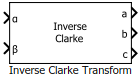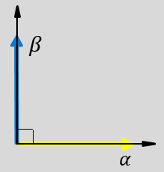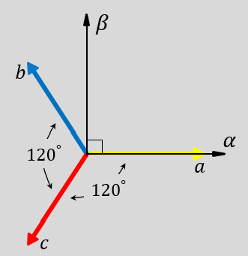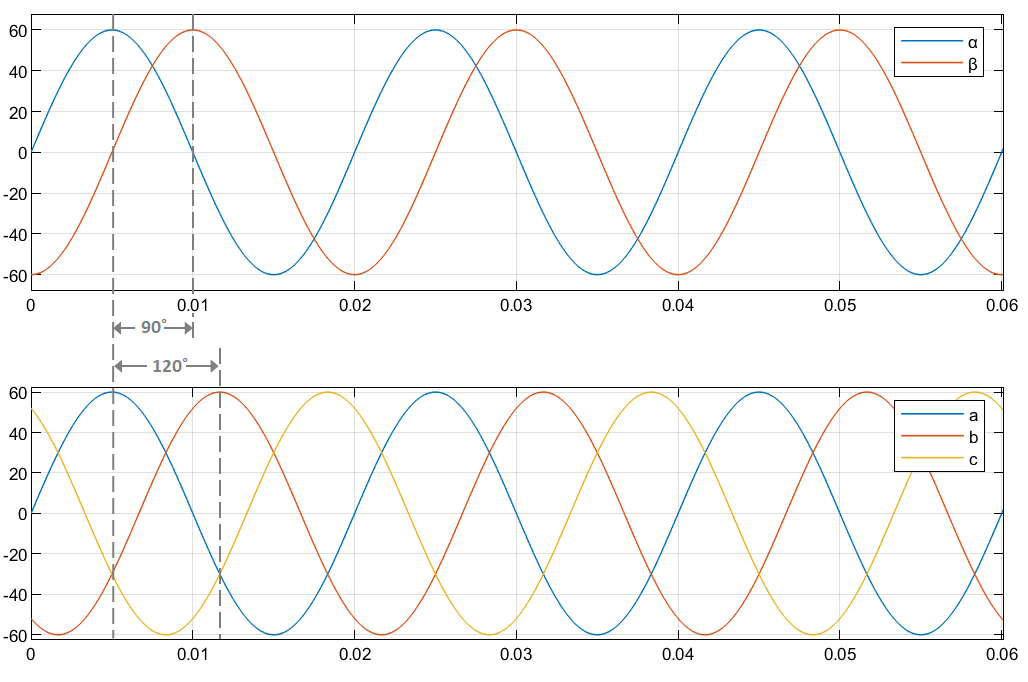# Inverse Clarke Transform

Implement αβ to abc transformation

Since R2020a

•Libraries:
Motor Control Blockset / Controls / Math Transforms
Motor Control Blockset HDL Support / Controls / Math Transforms

## Description

The Inverse Clarke Transform block computes the Inverse Clarke transformation of balanced, two-phase orthogonal components in the stationary αβ reference frame and outputs the balanced, three-phase components in the stationary abc reference frame. Alternatively, the block can compute Inverse Clarke transformation of the components α, β, and `0` to output the three-phase components a, b, and c. For a balanced system, the zero component is equal to zero. Use the Number of inputs parameter to use either two or three inputs.

The block accepts the α-β axis components as inputs and outputs the corresponding three-phase signals, where the phase-a axis aligns with the α-axis.

• The α and β input components in the αβ reference frame.• The direction of the equivalent a, b, and c output components in the abc reference frame and the αβ reference frame.• The time-response of the individual components of equivalent balanced αβ and abc systems.### Equations

The following equation describes the Inverse Clarke transform computation:

`$\left[\begin{array}{c}{f}_{a}\\ {f}_{b}\\ {f}_{c}\end{array}\right]=\left[\begin{array}{ccc}1& 0& 1\\ -\frac{1}{2}& \frac{\sqrt{3}}{2}& 1\\ -\frac{1}{2}& -\frac{\sqrt{3}}{2}& 1\end{array}\right]\left[\begin{array}{c}{f}_{\alpha }\\ {f}_{\beta }\\ {f}_{0}\end{array}\right]$`

For balanced systems like motors, the zero sequence component calculation is always zero:

`${i}_{a}+{i}_{b}+{i}_{c}=0$`

Therefore, you can use only two current sensors in three-phase motor drives, where you can calculate the third phase as,

`${i}_{c}=-\left({i}_{a}+{i}_{b}\right)$`

By using these equations, the block implements the Inverse Clarke transform as,

where:

• ${f}_{\alpha }$ and ${f}_{\beta }$ are the balanced two-phase orthogonal components in the stationary αβ reference frame.

• ${f}_{0}$ is the zero component in the stationary αβ reference frame.

• ${f}_{a}$, ${f}_{b}$, and ${f}_{c}$ are the balanced three-phase components in the abc reference frame.

## Ports

### Input

expand all

Alpha-axis component, α, in the stationary αβ reference frame.

#### Dependencies

To enable this port, set the Number of inputs parameter to `Two inputs`.

Data Types: `single` | `double` | `fixed point`

Beta-axis component, β, in the stationary αβ reference frame.

#### Dependencies

To enable this port, set the Number of inputs parameter to `Two inputs`.

Data Types: `single` | `double` | `fixed point`

Multiplexed alpha-axis component, α and beta-axis component, β and `0` component, in the stationary αβ reference frame.

#### Dependencies

To enable this port, set the Number of inputs parameter to `Three inputs`.

Data Types: `single` | `double` | `fixed point`

### Output

expand all

Component of the three-phase system in the abc reference frame.

#### Dependencies

To enable this port, set the Number of inputs parameter to `Two inputs`.

Data Types: `single` | `double` | `fixed point`

Component of the three-phase system in the abc reference frame.

#### Dependencies

To enable this port, set the Number of inputs parameter to `Two inputs`.

Data Types: `single` | `double` | `fixed point`

Component of the three-phase system in the abc reference frame.

#### Dependencies

To enable this port, set the Number of inputs parameter to `Two inputs`.

Data Types: `single` | `double` | `fixed point`

Multiplexed phase components a,b, and c of the three-phase system in the abc reference frame.

#### Dependencies

To enable this port, set the Number of inputs parameter to `Three inputs`.

Data Types: `single` | `double` | `fixed point`

## Parameters

expand all

Select the number of inputs that you can specify:

• `Two inputs` — Configure the block to accept two separate input signals α and β. The block generates three separate output signals a, b, and c.

• `Three inputs` — Configure the block to accept a multiplexed input containing α, β, and 0 signals. The block generates a multiplexed output containing a,b, and c signals.

To enable the power invariance property in the block output, select this parameter. To disable power invariance (enable amplitude invariance) in the block output, clear this parameter.

## Version History

Introduced in R2020a